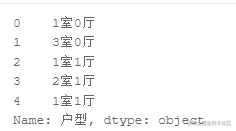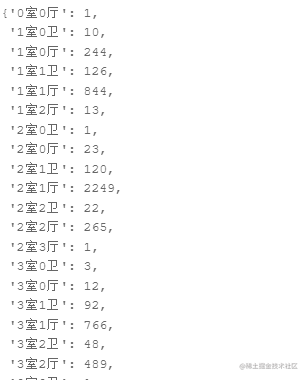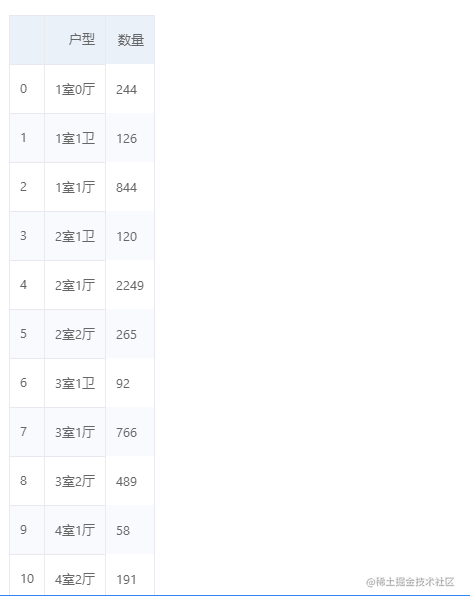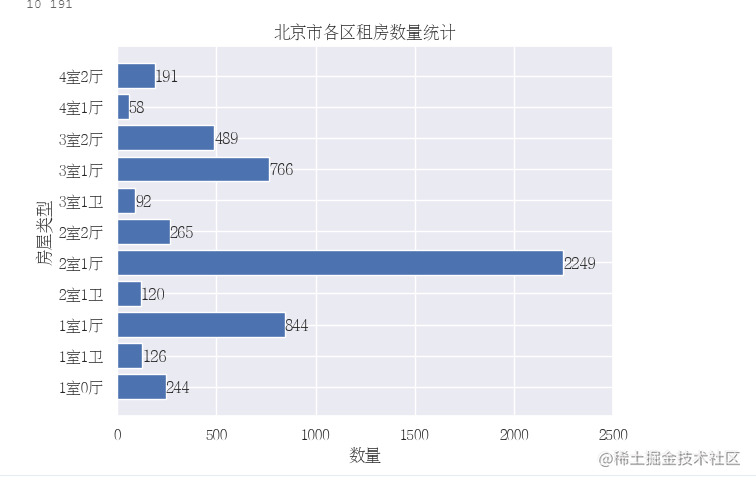# 2. 程式碼演示

## 2.1 戶型數量分析``````for k in key:

v = arr_new.size
result[k] = v

return result
``````

``` 呼叫函式寫入資料：

```python house_info = all_house(house_data) house_info``` 執行結果如下圖所示：# 篩選出大於50的資料

house_data = dict((key, value) for key, value in house_info.items() if value > 50) ``` 然後，將字典轉為表格形式： - 使用pd.DataFrame寫入資料

```python

# 字典轉為列表

show_houses = pd.DataFrame({"戶型":[x for x in house_data.keys()], "數量":[x for x in house_data.values()]}) show_houses ``` 執行結果如下圖所示：## 2.2 戶型數量視覺化

• plt.title：為影象新增名稱
• 前三行程式碼表示開啟支援中文顯示
• y+0.5,x-0.2：調節柱上數字顯示位置 ```python from matplotlib.font_manager import FontProperties myfont=FontProperties(fname=r'/usr/share/fonts/fangzheng/FZSYJW.TTF',size=12) sns.set(font=myfont.get_name())

# 圖形展示：房屋型別

house_type = show_houses["戶型"] house_type_num = show_houses["數量"] plt.barh(range(11), house_type_num) plt.yticks(range(11), house_type) plt.xlim(0,2500) plt.title("北京市各區租房數量統計")

plt.xlabel("數量") plt.ylabel("房屋型別")

# 給每個條上面新增具體數字

for x,y in enumerate(house_type_num): print(x,y) plt.text(y+0.5,x-0.2,"%s" %y) plt.show() ``` 執行結果如下圖所示：